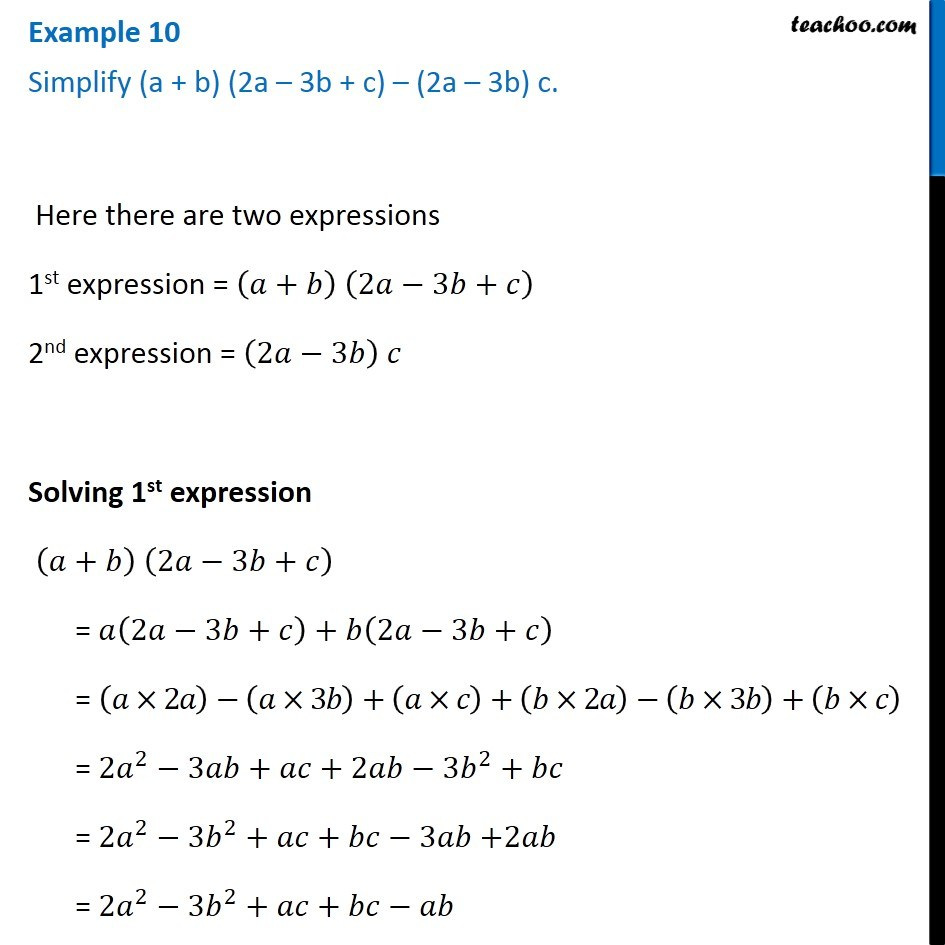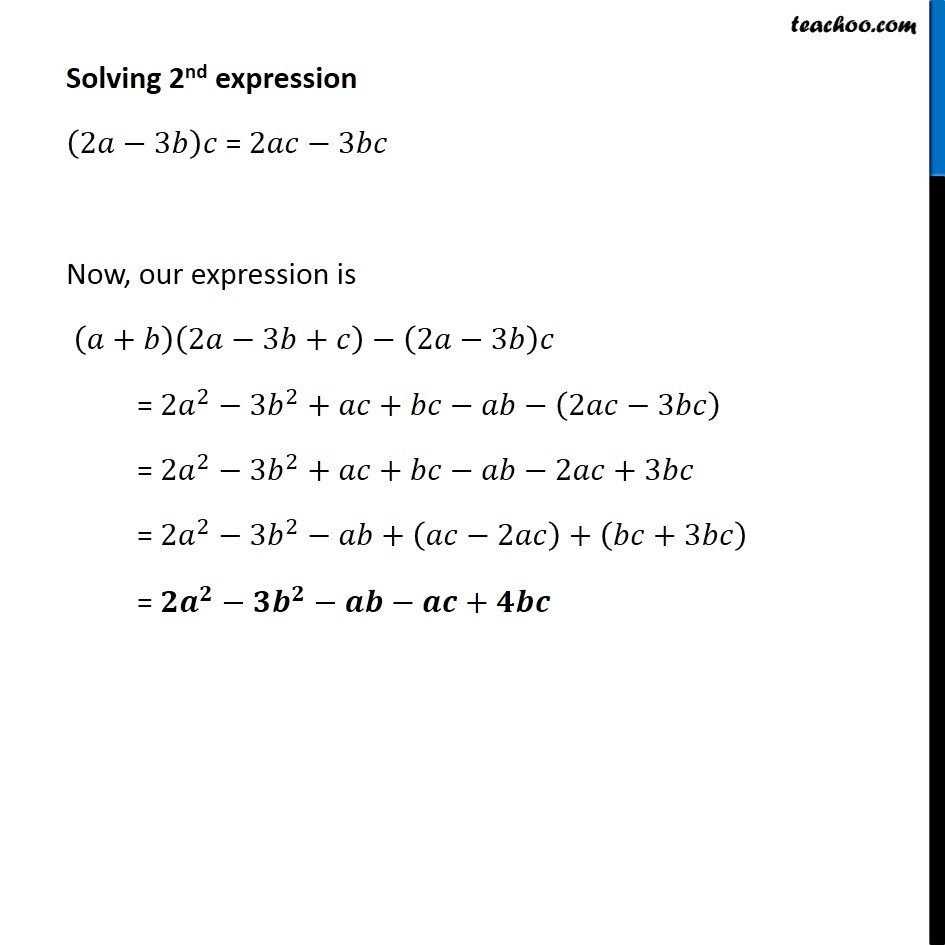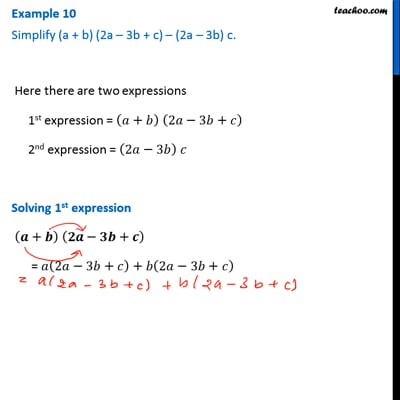Examples

Chapter 8 Class 8 Algebraic Expressions and Identities
Serial order wiseThis video is only available for Teachoo black users

Learn in your speed, with individual attention - Teachoo Maths 1-on-1 Class

### Transcript

Example 10 Simplify (a + b) (2a – 3b + c) – (2a – 3b) c. Here there are two expressions 1st expression = (𝑎+𝑏) (2𝑎−3𝑏+𝑐) 2nd expression = (2𝑎−3𝑏) 𝑐 Solving 1st expression (𝑎+𝑏) (2𝑎−3𝑏+𝑐) = 𝑎(2𝑎−3𝑏+𝑐)+𝑏(2𝑎−3𝑏+𝑐) = (𝑎×2𝑎)−(𝑎×3𝑏)+(𝑎×𝑐)+(𝑏×2𝑎)−(𝑏×3𝑏)+(𝑏×𝑐) = 2𝑎^2−3𝑎𝑏+𝑎𝑐+2𝑎𝑏−3𝑏^2+𝑏𝑐 = 2𝑎^2−3𝑏^2+𝑎𝑐+𝑏𝑐−3𝑎𝑏 +2𝑎𝑏 = 2𝑎^2−3𝑏^2+𝑎𝑐+𝑏𝑐−𝑎𝑏 Solving 2nd expression (2𝑎−3𝑏)𝑐 = 2𝑎𝑐−3𝑏𝑐 Now, our expression is (𝑎+𝑏)(2𝑎−3𝑏+𝑐)−(2𝑎−3𝑏)𝑐 = 2𝑎^2−3𝑏^2+𝑎𝑐+𝑏𝑐−𝑎𝑏−(2𝑎𝑐−3𝑏𝑐) = 2𝑎^2−3𝑏^2+𝑎𝑐+𝑏𝑐−𝑎𝑏−2𝑎𝑐+3𝑏𝑐 = 2𝑎^2−3𝑏^2−𝑎𝑏+(𝑎𝑐−2𝑎𝑐)+(𝑏𝑐+3𝑏𝑐) = 𝟐𝒂^𝟐−𝟑𝒃^𝟐−𝒂𝒃−𝒂𝒄+𝟒𝒃𝒄